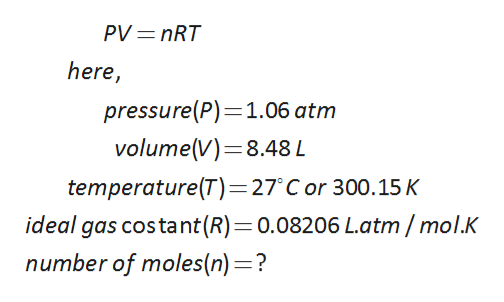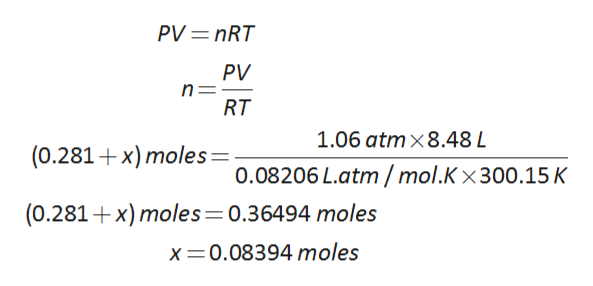# A mixture consisting of 0.140 mol N2, 0.037 mol O2, 0.104 mol CH4, and an unknown amount of CO2 occupies a volume of 8.48 L at 27°C and 1.06 atm pressure. How many moles of CO2 are there in this sample?a. 0.719 molb. 2.45 molc. 0.364 mold. 0.0839 mole. 3.77 mol

Question
6 views

A mixture consisting of 0.140 mol N2, 0.037 mol O2, 0.104 mol CH4, and an unknown amount of CO2 occupies a volume of 8.48 L at 27°C and 1.06 atm pressure. How many moles of CO2 are there in this sample?

a. 0.719 mol

b. 2.45 mol

c. 0.364 mol

d. 0.0839 mol

e. 3.77 mol

check_circle

Step 1

To calculate the moles of carbon dioxide of given sample using the following gas law formula.help_outlineImage TranscriptionclosePV=nRT here, pressure(P)-1.06 atm volume(V) 8.48 L temperature(T)=27'C or 300.15K ideal gas costant(R)=0.08206 L.atm/ mol.K number of moles(n)=? fullscreen
Step 2

Let us take x is the moles of carbon dioxide, then the total moles of the givne sample as follows:

Step 3

The calculation of moles of carbon diox...help_outlineImage TranscriptionclosePV=nRT PV RT 1.06 atmx8.48 L (0.281 x) moles=. 0.08206 L.atm/mol.K x 300. 15 K (0.281 x) moles=0.36494 moles x 0.08394 moles fullscreen

### Want to see the full answer?

See Solution

#### Want to see this answer and more?

Solutions are written by subject experts who are available 24/7. Questions are typically answered within 1 hour.*

See Solution
*Response times may vary by subject and question.
Tagged in

### Chemistry